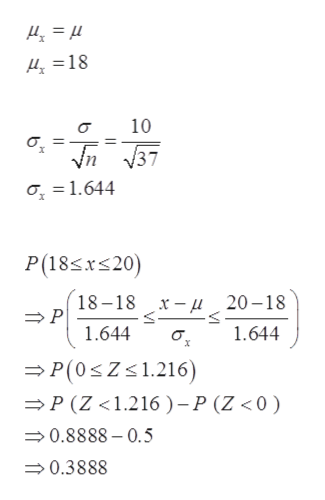# Suppose x has a distribution with μ = 18 and σ = 10.(a) If a random sample of size n = 37 is drawn, find μx, σ x and P(18 ≤ x ≤ 20). (Round σx to two decimal places and the probability to four decimal places.)μx =  σ x =  P(18 ≤ x ≤ 20) =  (b) If a random sample of size n = 68 is drawn, find μx, σ x and P(18 ≤ x ≤ 20). (Round σ x to two decimal places and the probability to four decimal places.)μx =  σ x =  P(18 ≤ x ≤ 20) =

Question
65 views

Suppose x has a distribution with μ = 18 and σ = 10.

(a) If a random sample of size n = 37 is drawn, find μx, σ x and P(18 ≤ x ≤ 20). (Round σx to two decimal places and the probability to four decimal places.)
 μx = σ x = P(18 ≤ x ≤ 20) =

(b) If a random sample of size n = 68 is drawn, find μx, σ x and P(18 ≤ x ≤ 20). (Round σ x to two decimal places and the probability to four decimal places.)
 μx = σ x = P(18 ≤ x ≤ 20) =
check_circle

Step 1

In this question, it has been given that X follows a distribution with mean 18 and standard deviation 10.

Step 2

Part a)

n = 37

Now, Probability P (18 ≤&nbs...help_outlineImage Transcriptioncloseri = *r1 18 10 уn у37 1.644 P(18sxs20) 18-18 х — д 20—18 P 1.644 1.644 P(0Z1.216) P (Z <1.216 ) -P (Z <0) 0.8888-0.5 0.3888 fullscreen

### Want to see the full answer?

See Solution

#### Want to see this answer and more?

Solutions are written by subject experts who are available 24/7. Questions are typically answered within 1 hour.*

See Solution
*Response times may vary by subject and question.
Tagged in

### Other